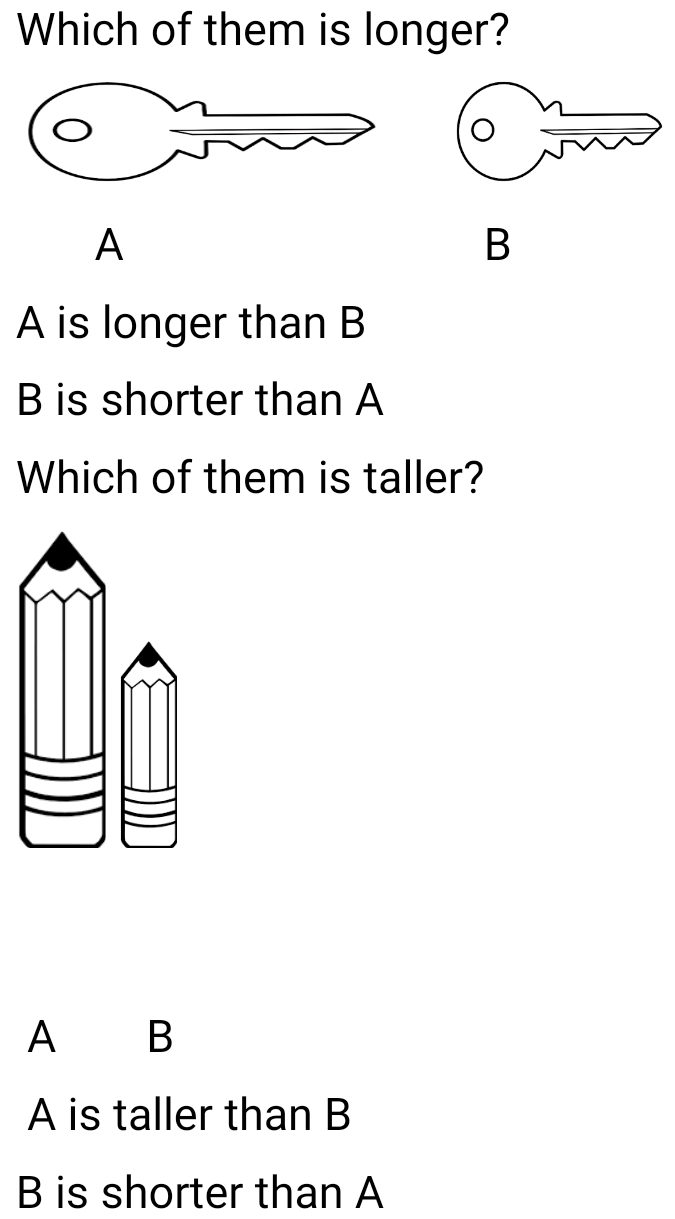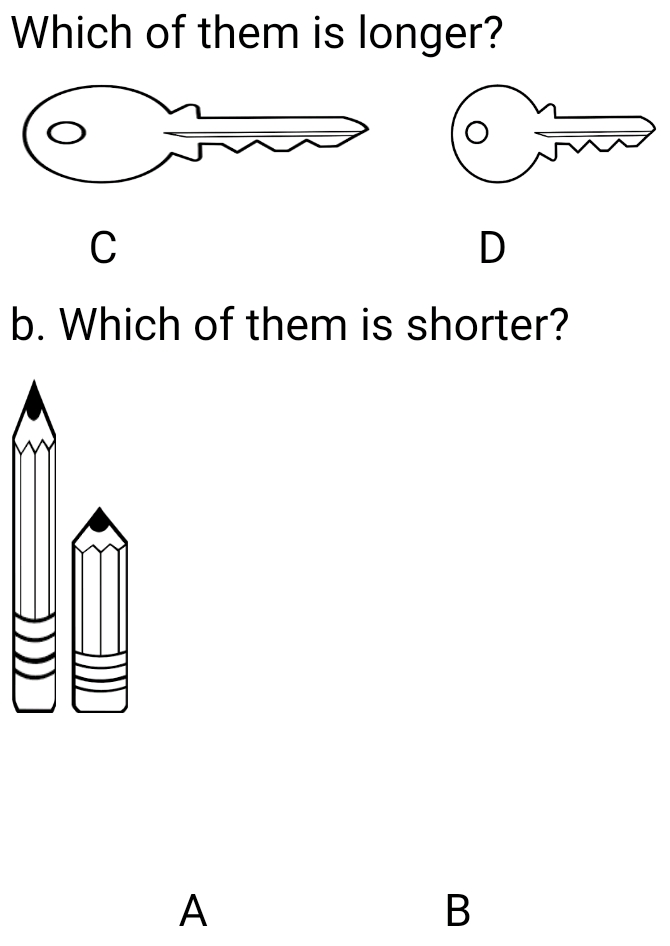# Lesson Notes By Weeks and Term - Nursery 2

Counting/identification of numbers 1 - 550

SUBJECT: Numeracy

TERM: 3rd Term

Week: 1

CLASS – Nursery 2

AGE – 5 years

DURATION – 5 periods of 35 minutes each

TOPIC- Counting/identification of numbers 1-550

CONTENT – Writing 250-300, Number endings. Measurement of length and height. 5x table. Sensorial-arranging like shapes using beads

INSTRUCTIONAL OBJECTIVES – By the end of the lesson, the pupils should be able to –

1. Count and identify numbers 1-550
2. Write numbers 250-300
3. Recite the 5x table
4. Determine which is longer/taller between two objects

INSTRUCTIONAL MATERIALS – flash cards, charts, counters

INSTRUCTIONAL TECHNIQUES – Explanation, repetition, questions and answers

ENTRY BEHAVIOUR – The pupils can count numbers 1-500 and spell numbers 1-60

SET INDUCTION –She uses number rhyme to arouse their interest

INSTRUCTIONAL PROCEDURE

PERIOD I: Counting numbers 1-550 and writing 250-300

 STEPS TEACHER’S ACTIVITY PUPILS’ ACTIVITY IIntroduction The teacher reviews the previous lesson introduces the new topic Pupils participate IICounting Using the number chart, she counts number 1-550 repeatedly. Pupils repeat after her IIIWriting numbers She counts and write numbers 250-300 on the board; she also uses flashcards Pupils count and write in their book IVNumber ending She writes the number endings on the board and also uses flashcards Pupils count Evaluation Count and write numbers 250-300 Conclusion She marks their books and corrects them where necessary

PERIOD II& III: Measurement of length and height, 5x times table

 STEPS TEACHER’S ACTIVITY PUPILS’ ACTIVITY IIntroduction The teacher reviews the previous lesson introduces the new topic Pupils participate IIMeasurement of length/height She tells them that length is how long or short something is and height is how tall or short an object is.Example:Which of them is longer?Pupils identify tall and short object III5x table She writes the 5x table on the board and recites it severally as the pupils recite after her5 x 1 = 55 x 2 = 105 x 3 = 155 x 4 = 205 x 5 = 255 x 6 = 305 x 7 = 355 x 8 = 405 x 9 = 455 x 10 = 505 x 11 = 555 x 12 = 60 Evaluation 1a.2. Complete the 5x table5 x 1 =5 x 2 =5 x 3 =5 x 4 = Conclusion She marks their books and corrects them where necessary

PERIOD V: Sensorial- arranging beads according to their shapes

 STEPS TEACHER’S ACTIVITY PUPILS’ ACTIVITY IIntroduction The teacher reviews the basic shapes and introduces the new topic Pupils respond IISorting beads according to shapes The teacher divides the pupils into four groups. Each group is to pick out the triangle, square, rectangle and circle respectively Pupils sort out the beads into shapes Evaluation The pupils are scored based on how well each group sorted out the beads into shapes Conclusion She marks their books and corrects them where necessary